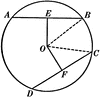# Equal Chords in Equal Circles TheoremIllustration used to show that “In equal circles, or in the same circle, if two chords are equal, they are equally distant from the center; conversely, if two chords are equally distant from the center, they are equal.”

### Keywords

geometry, circle, arc, proof, chord, chords, theorem, equal arcs, prove, theorems, proofs

### Galleries

Geometric Theorems and Proofs

### Source

C.A. Hart, Daniel D. Feldman Plane and Solid Geometry (New York, NY: American Book Company, 1911)

TIFF (full resolution)

2400×2336, 304.3 KiB

Large GIF

1024×996, 37.3 KiB

Medium GIF

640×622, 20.2 KiB

Small GIF

320×311, 8.8 KiB